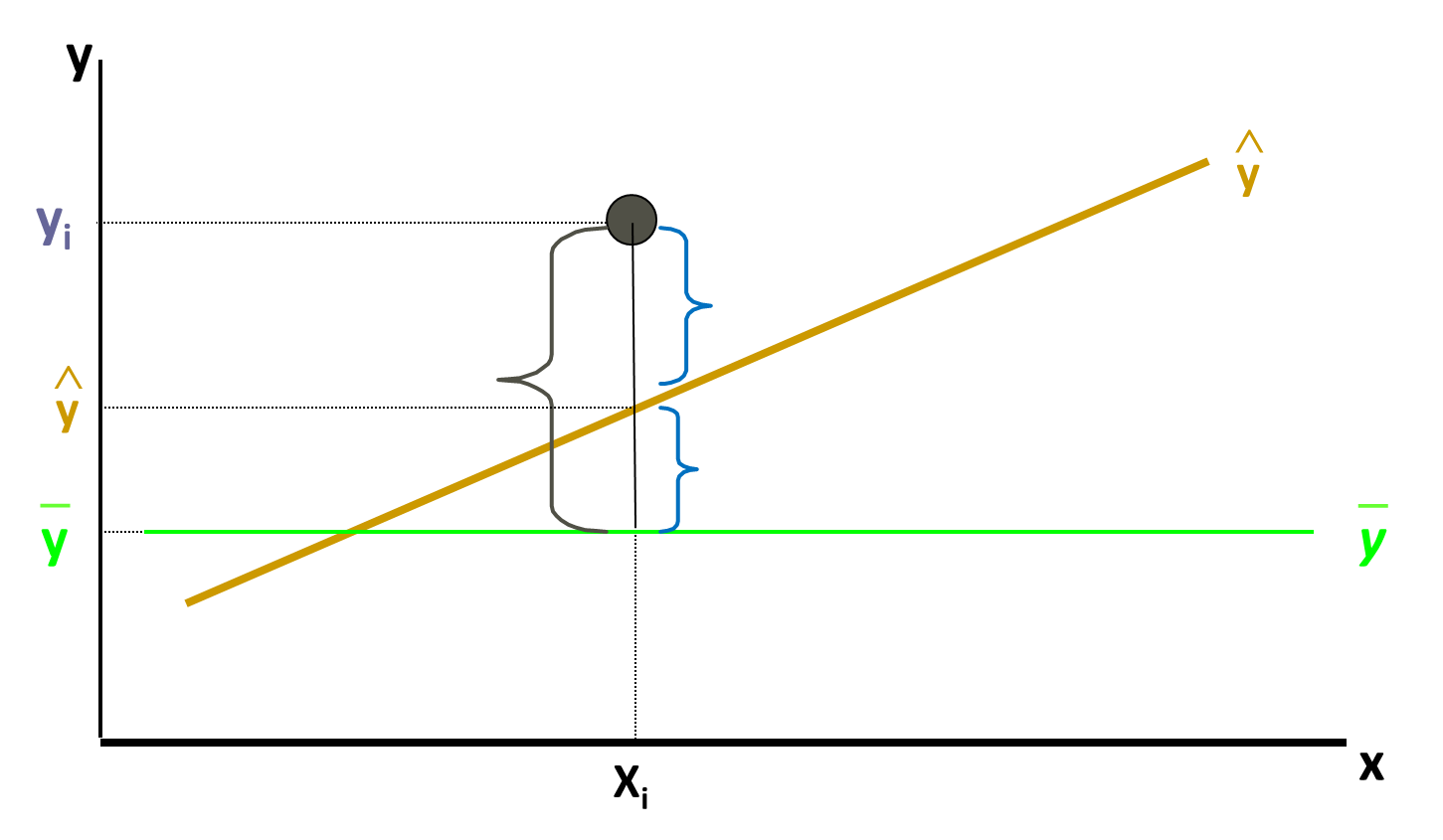• No products in the cart.

# 204.1.4 How good is my Regression Line

##### Mathematics behind a good fit.

Link to the previous post : https://statinfer.com/204-1-3-practice-regression-line-fitting/

In this post we will understand the mathematics behind a good regression line.

### How good is my regression line?

• Take an (x,y) point from data.
• Imagine that we submitted x in the regression line, we got a prediction as ypred
• If the regression line is a good fit then the we expect ypred=y or (y-ypred) =0
• At every point of x, if we repeat the same, then we will get multiple error values (y-ypred) values
• Some of them might be positive, some of them may be negative, so we can take the square of all such errors
SSE=(yy^)2
• For a good model we need SSE to be zero or near to zero
• Standalone SSE will not make any sense, For example SSE= 100, is very less when y is varying in terms of 1000’s. Same value is is very high when y is varying in terms of decimals.
• We have to consider variance of y while calculating the regression line accuracy
• Error Sum of squares (SSE- Sum of Squares of error)
SSE=(yy^)2
• Total Variance in Y (SST- Sum of Squares of Total)
SST=(yy¯)2
SST=(yy^+y^y¯)2
SST=(yy^+y^y¯)2
SST=(yy^)2+(y^y¯)2
SST=SSE+(y^y¯)2
SST=SSE+SSR
• So, total variance in Y is divided into two parts,
• Variance that can’t be explained by x (error)
• Variance that can be explained by x, using regression

### Explained and Unexplained Variation• Total variance in Y is divided into two  parts,
• Variance that can be explained by x, using regression
• Variance that can’t be explained by x
SST=SSE+SSR
TotalsumofSquares=SumofSquaresError+SumofSquaresRegression
SST=(yy¯)2SSE=(yy^)2SSR=(y^y¯)2

In next session we will figure out Rsquared which a statistical measure of closeness of datapoints to the fitted regression line.

The next post is about R squared in python.

Link to the next post : https://statinfer.com/204-1-5-r-squared-in-python/

21st June 2017